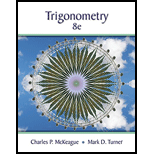Chapter 1.4, Problem 22PSTrigonometry (MindTap Course List)

8th Edition
Charles P. McKeague + 1 other
ISBN: 9781305652224

Solutions

Chapter
SectionTrigonometry (MindTap Course List)

8th Edition
Charles P. McKeague + 1 other
ISBN: 9781305652224
Textbook Problem

Use a ratio identity to find tan   θ given the following values. sin   θ = 3 5 and cos   θ = − 4 5

To determine

To find the value of the given expression using ratio identity.

Explanation

Given:

sinθ=35 and cosθ=45.

Concept used:

The ratio identity of trigonometric functions.

Calculation:

We know, cotθ=cosθsinθ.

Substitute the given values in the above identity and simplify.

cotθ=cosθsinθ=

Still sussing out bartleby?

Check out a sample textbook solution.

See a sample solution

The Solution to Your Study Problems

Bartleby provides explanations to thousands of textbook problems written by our experts, many with advanced degrees!

Get Started

Under what circumstances is a t statistic used instead of a z-score for a hypothesis test?

Essentials of Statistics for The Behavioral Sciences (MindTap Course List)

Find all possible real solutions of each equation in Exercises 3144. x3x25x+5=0

Finite Mathematics and Applied Calculus (MindTap Course List)

Simplify: j26

Elementary Technical Mathematics

If f(t)=4t+1, find f"(2).

Calculus (MindTap Course List)

The graph at the right has a local maximum at x ______. a) 1, 3, and 5 b) 2 c) 4 d) 2 and 4

Study Guide for Stewart's Single Variable Calculus: Early Transcendentals, 8th

True or False: is a geometric series.

Study Guide for Stewart's Multivariable Calculus, 8th